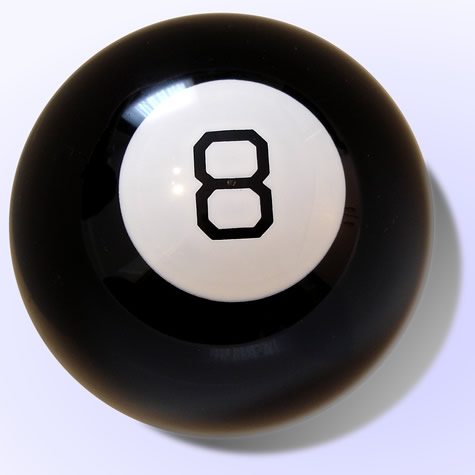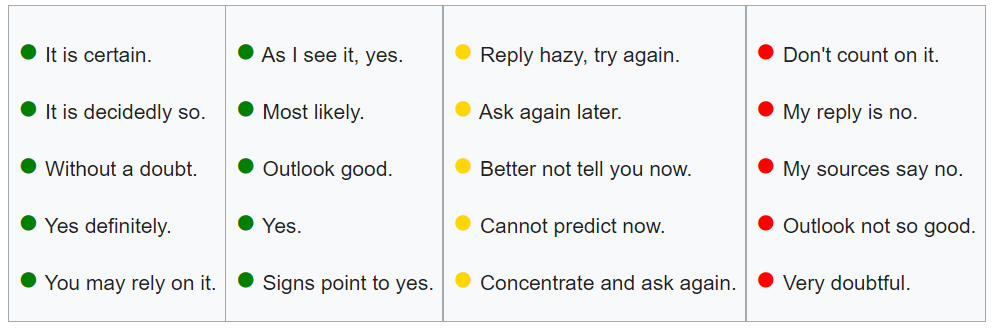# Stochastic Function: Definition, Examples

## What is a Stochastic Function?

A stochastic function is a function with an uncertain input . One or more of the function’s parameters has a term with statistical noise.

A stochastic function can also be described as an ensemble of continuous functions used to model random behavior. If a model is a stochastic function, it means giving probabilities for various sets of functions with different local properties of sampling functions (like smoothness) and distributions of functionals (like minimums) .

## Deterministic vs. Stochastic Functions

In many ways, a stochastic function can be thought of as the opposite of a deterministic function. A deterministic function will give you the same result over and over again, given the same inputs. On the other hand, a stochastic function will give you different results, given those same initial inputs. As a simple example, addition of numbers is a deterministic function: Assuming you’re working in base 10, 4 plus 2 always equals 6 no matter how many times you complete the calculation.

As another example of a deterministic function, let’s say you had a pool ball that was heavily weighted and always landed on the number 8.Every time you roll the ball, no matter how hard or in what direction, you always get the same result: 8. You could model this with a deterministic function that would look something like this:

Roll 8 Ball = 8.

On the other hand, the magic 8 ball is a fortune telling ball that gives one of 20 answers, each of which has an equal probability. Every time you shake the ball, you have a 1/20 chance of getting any of the 20 possible responses:Given identical conditions (shaking the ball from left to right or up and down), the ball will not return the same value. You might get a “Yes definitely”, a “Cannot predict now” or a “Don’t count on it,” even though you do your best to be repeatable. The ball does not consistently return the same value, but you can calculate the probabilities of each answer (in this case, 1/20). Therefore, the Magic 8 Ball can be modeled with a stochastic function.

## References

 Wojnowicz, M. (2012). The Ornstein-Uhlenbeck Process In Neural Decision-Making: Mathematical Foundations And Simulations Suggesting The Adaptiveness Of Robustly Integrating Stochastic Neural Evidence. Retrieved September 28, 2021 from: https://digital.lib.washington.edu/researchworks/bitstream/handle/1773/21760/Wojnowicz_washington_0250O_10917.pdf?sequence=1
 Telksnys, L. Stochastic Control. Proceedings of the 2nd IFAC Symposium, Vilnius, Lithuanian SSR, USSR, 19-23 May 1986

CITE THIS AS:
Stephanie Glen. "Stochastic Function: Definition, Examples" From StatisticsHowTo.com: Elementary Statistics for the rest of us! https://www.statisticshowto.com/stochastic-function/